# 前言

DirectX11 With Windows SDK完整目录

Github项目源码

# 法线贴图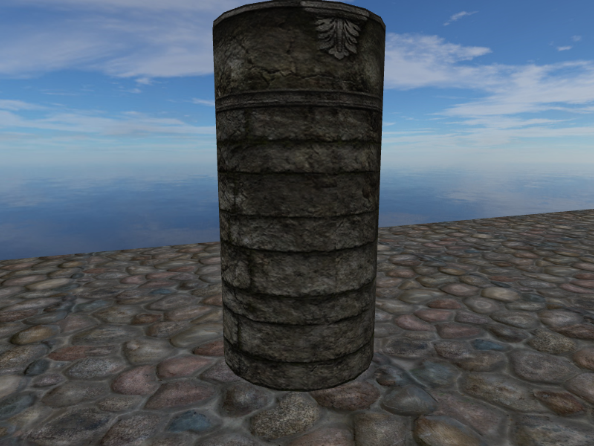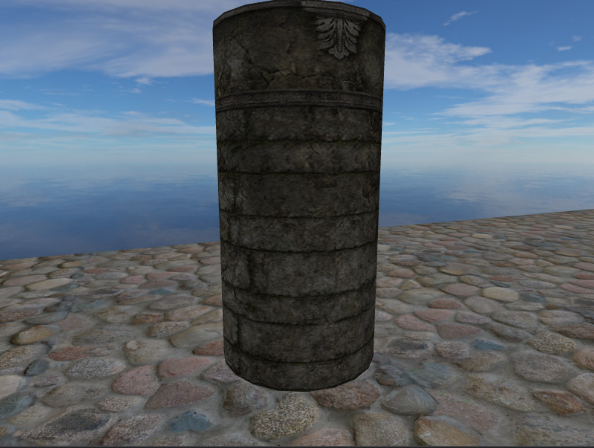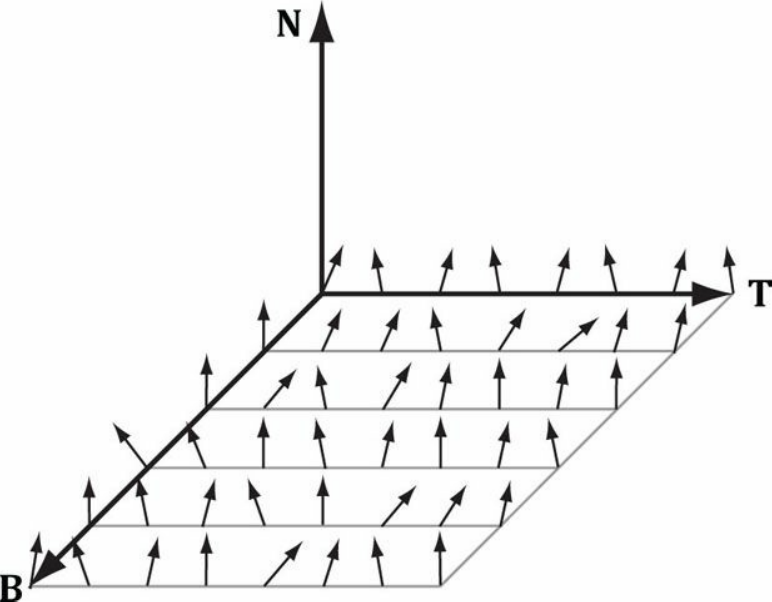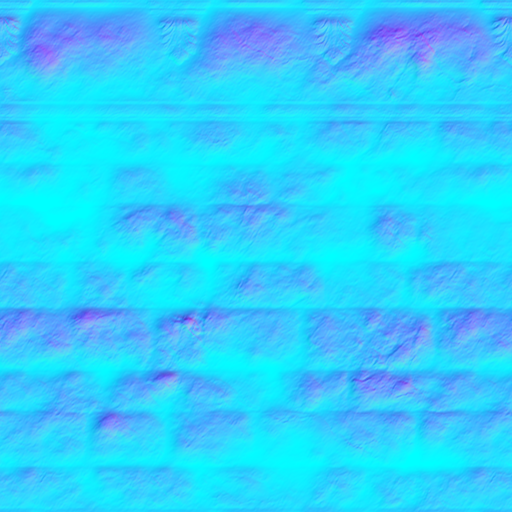## 法线贴图的压缩与解压

$f(x) = (0.5x + 0.5) * 255$

$f^-1(x) = \frac{2x}{255} - 1$

float3 normalT = gNormalMap.Sample(sam, pin.Tex);


normalT = 2.0f * normalT - 1.0f;


# 纹理/切线空间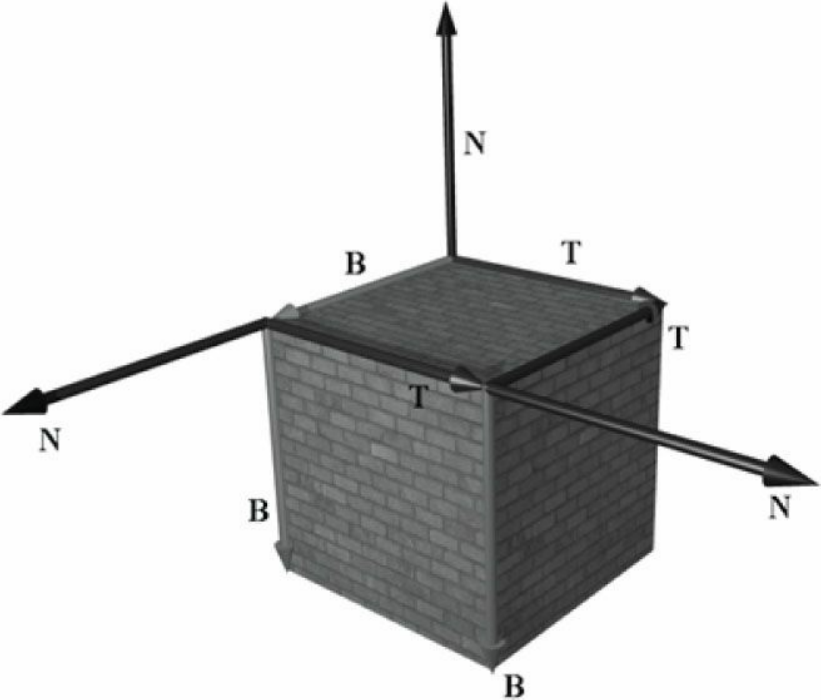## 利用顶点位置和纹理坐标求TBN坐标系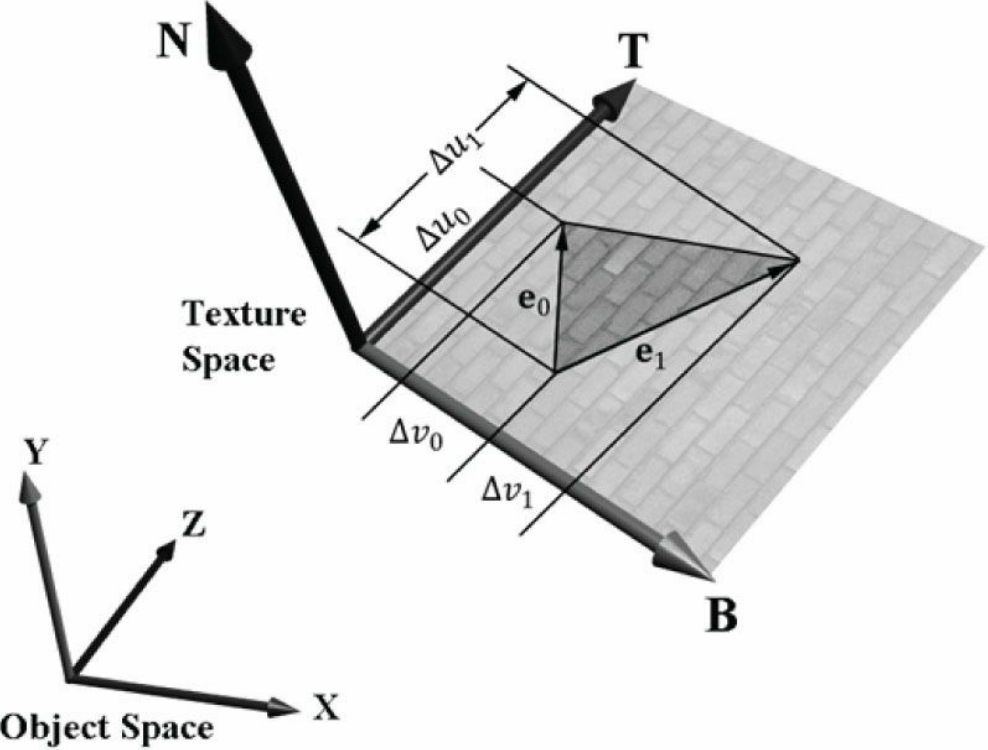$\mathbf{e_0} = \mathbf{V_1} - \mathbf{V_0} \\ \mathbf{e_1} = \mathbf{V_2} - \mathbf{V_0}$

$(\Delta u_0, \Delta v_0) = (u_1 - u_0, v_1 - v_0) \\ (\Delta u_1, \Delta v_1) = (u_2 - u_0, v_2 - v_0) \\ \mathbf{e_0} = \Delta u_0\mathbf{T} + \Delta v_0\mathbf{B} \\ \mathbf{e_1} = \Delta u_1\mathbf{T} + \Delta v_1\mathbf{B}$

$\begin{bmatrix} \mathbf{e_0} \\ \mathbf{e_1} \end{bmatrix} = \begin{bmatrix} \Delta u_0 & \Delta v_0 \\ \Delta u_1 & \Delta v_1 \end{bmatrix} \begin{bmatrix} \mathbf{T} \\ \mathbf{B} \end{bmatrix}$

$\begin{bmatrix} e_{0x} & e_{0y} & e_{0z} \\ e_{1x} & e_{1y} & e_{1z} \end{bmatrix} = \begin{bmatrix} \Delta u_0 & \Delta v_0 \\ \Delta u_1 & \Delta v_1 \end{bmatrix} \begin{bmatrix} T_x & T_y & T_z \\ B_x & B_y & B_z \end{bmatrix}$

${\begin{bmatrix} \Delta u_0 & \Delta v_0 \\ \Delta u_1 & \Delta v_1 \end{bmatrix}}^{-1} \begin{bmatrix} e_{0x} & e_{0y} & e_{0z} \\ e_{1x} & e_{1y} & e_{1z} \end{bmatrix} = \begin{bmatrix} T_x & T_y & T_z \\ B_x & B_y & B_z \end{bmatrix}$

$\mathbf{A}^{-1} = \frac{1}{ad-bc}\begin{bmatrix} d & -b \\ -c & a \end{bmatrix}$

$\begin{bmatrix} T_x & T_y & T_z \\ B_x & B_y & B_z \end{bmatrix} = \frac{1}{\Delta u_0 \Delta v_1 - \Delta v_0 \Delta u_1} \begin{bmatrix} \Delta v_1 & - \Delta v_0 \\ -\Delta u_1 & \Delta u_0 \end{bmatrix} \begin{bmatrix} e_{0x} & e_{0y} & e_{0z} \\ e_{1x} & e_{1y} & e_{1z} \end{bmatrix}$

V0坐标为(0, 0, -0.25), 纹理坐标为(0, 0.5)
V1坐标为(0.15, 0, 0), 纹理坐标为(0.3, 0)
V2坐标为(0.4, 0, 0), 纹理坐标为(0.8, 0)

\begin{align}\mathbf{e_0} &= \mathbf{V_1} - \mathbf{V_0} = (0.15, 0, 0.25) \\ \mathbf{e_1} &= \mathbf{V_2} - \mathbf{V_0} = (0.4, 0, 0.25) \\ (\Delta u_0, \Delta v_0) &= (u_1 - u_0, v_1 - v_0) = (0.3, -0.5) \\ (\Delta u_1, \Delta v_1) &= (u_2 - u_0, v_2 - v_0) = (0.8, -0.5) \\ \begin{bmatrix} T_x & T_y & T_z \\ B_x & B_y & B_z \end{bmatrix} &= \frac{1}{0.3 \times (-0.5) - (-0.5) \times 0.8} \begin{bmatrix} -0.5 & 0.5 \\ -0.8 & 0.3 \end{bmatrix} \begin{bmatrix} 0.15 & 0 & 0.25 \\ 0.4 & 0 & 0.25 \end{bmatrix} = \begin{bmatrix} 0.5 & 0 & 0 \\ 0 & 0 & -0.5 \end{bmatrix}\end{align}

# 顶点切线空间

struct VertexPosNormalTangentTex
{
DirectX::XMFLOAT3 pos;
DirectX::XMFLOAT3 normal;
DirectX::XMFLOAT4 tangent;
DirectX::XMFLOAT2 tex;
static const D3D11_INPUT_ELEMENT_DESC inputLayout;
};


## 施密特向量正交化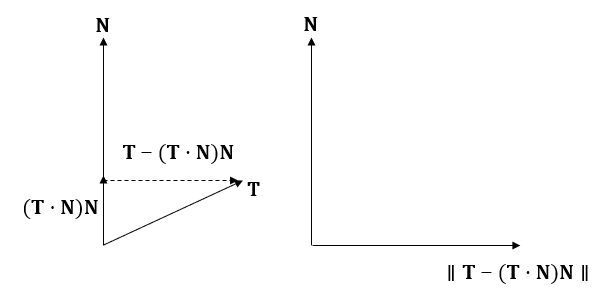$\mathbf{T'} = \lVert \mathbf{T} - (\mathbf{T} \cdot \mathbf{N}) \mathbf{N} \rVert$

B' 最终也可以确定下来

$\mathbf{B'} = \mathbf{N} \times \mathbf{T'}$

## 切线空间的变换

$\mathbf{M}_{object} = \begin{bmatrix} T_x & T_y & T_z \\ B_x & B_y & B_z \\ N_x & N_y & N_z \end{bmatrix}$

$\mathbf{n}_{world} = \mathbf{n}_{tangent}\mathbf{M}_{object}\mathbf{M}_{world}$

1. 对切线向量进行矩阵变换，我们只需要使用3x3的矩阵即可。
2. 法线向量变换到世界矩阵需要用世界矩阵求逆的转置进行校正，而对切线向量只需要用世界矩阵变换即可。下图演示了将宽度拉伸为原来2倍后，法线和切线向量的变化：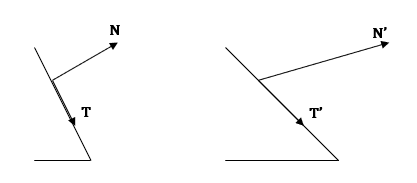# HLSL代码

1. 获取该纹理所需要用到的法线贴图，在C++端为其创建一个ID3D11Texture2D。这里不考虑如何制作一张法线贴图。
2. 对于一个网格模型来说，顶点数据需要包含位置、法向量、切线向量、纹理坐标四个元素。同样这里不讨论模型的制作，在本教程使用的是Geometry所生成的网格模型
3. 在顶点着色器中，将顶点法向量和切线向量从局部坐标系变换到世界坐标系
4. 在像素着色器中，使用经过插值的法向量和切线向量来为每个三角形表面的像素点构建TBN坐标系，然后将切线空间的法向量变换到世界坐标系中，这样最终求得的法向量用于光照计算。

Texture2D g_DiffuseMap : register(t0);
Texture2D g_NormalMap : register(t1);
TextureCube g_TexCube : register(t2);
SamplerState g_Sam : register(s0);

// 使用的是第23章的常量缓冲区，省略...
// 省略和之前一样的结构体...

struct VertexPosNormalTangentTex
{
float3 PosL : POSITION;
float3 NormalL : NORMAL;
float4 TangentL : TANGENT;
float2 Tex : TEXCOORD;
};

struct InstancePosNormalTangentTex
{
float3 PosL : POSITION;
float3 NormalL : NORMAL;
float4 TangentL : TANGENT;
float2 Tex : TEXCOORD;
matrix World : World;
matrix WorldInvTranspose : WorldInvTranspose;
};

struct VertexPosHWNormalTangentTex
{
float4 PosH : SV_POSITION;
float3 PosW : POSITION; // 在世界中的位置
float3 NormalW : NORMAL; // 法向量在世界中的方向
float4 TangentW : TANGENT; // 切线在世界中的方向
float2 Tex : TEXCOORD;
};

float3 NormalSampleToWorldSpace(float3 normalMapSample,
float3 unitNormalW,
float4 tangentW)
{
// 将读取到法向量中的每个分量从[0, 1]还原到[-1, 1]
float3 normalT = 2.0f * normalMapSample - 1.0f;

// 构建位于世界坐标系的切线空间
float3 N = unitNormalW;
float3 T = normalize(tangentW.xyz - dot(tangentW.xyz, N) * N); // 施密特正交化
float3 B = cross(N, T);

float3x3 TBN = float3x3(T, B, N);

// 将凹凸法向量从切线空间变换到世界坐标系
float3 bumpedNormalW = mul(normalT, TBN);

return bumpedNormalW;
}


// NormalMapObject_VS.hlsl
#include "Basic.hlsli"

// 顶点着色器
VertexPosHWNormalTangentTex VS(VertexPosNormalTangentTex vIn)
{
VertexPosHWNormalTangentTex vOut;

matrix viewProj = mul(g_View, g_Proj);
vector posW = mul(float4(vIn.PosL, 1.0f), g_World);

vOut.PosW = posW.xyz;
vOut.PosH = mul(posW, viewProj);
vOut.NormalW = mul(vIn.NormalL, (float3x3) g_WorldInvTranspose);
vOut.TangentW = mul(vIn.TangentL, g_World);
vOut.Tex = vIn.Tex;
return vOut;
}

// NormalMapInstance_VS.hlsl
#include "Basic.hlsli"

// 顶点着色器
VertexPosHWNormalTangentTex VS(InstancePosNormalTangentTex vIn)
{
VertexPosHWNormalTangentTex vOut;

matrix viewProj = mul(g_View, g_Proj);
vector posW = mul(float4(vIn.PosL, 1.0f), vIn.World);

vOut.PosW = posW.xyz;
vOut.PosH = mul(posW, viewProj);
vOut.NormalW = mul(vIn.NormalL, (float3x3) vIn.WorldInvTranspose);
vOut.TangentW = mul(vIn.TangentL, vIn.World);
vOut.Tex = vIn.Tex;
return vOut;
}


// 法线映射
float3 normalMapSample = g_NormalMap.Sample(g_Sam, pIn.Tex).rgb;
float3 bumpedNormalW = NormalSampleToWorldSpace(normalMapSample, pIn.NormalW, pIn.TangentW);



// NormalMap_PS.hlsl
#include "Basic.hlsli"

// 像素着色器(3D)
float4 PS(VertexPosHWNormalTangentTex pIn) : SV_Target
{
// 若不使用纹理，则使用默认白色
float4 texColor = float4(1.0f, 1.0f, 1.0f, 1.0f);

if (g_TextureUsed)
{
texColor = g_DiffuseMap.Sample(g_Sam, pIn.Tex);
// 提前进行裁剪，对不符合要求的像素可以避免后续运算
clip(texColor.a - 0.1f);
}

// 标准化法向量
pIn.NormalW = normalize(pIn.NormalW);

// 求出顶点指向眼睛的向量，以及顶点与眼睛的距离
float3 toEyeW = normalize(g_EyePosW - pIn.PosW);
float distToEye = distance(g_EyePosW, pIn.PosW);

// 法线映射
float3 normalMapSample = g_NormalMap.Sample(g_Sam, pIn.Tex).rgb;
float3 bumpedNormalW = NormalSampleToWorldSpace(normalMapSample, pIn.NormalW, pIn.TangentW);

// 初始化为0
float4 ambient = float4(0.0f, 0.0f, 0.0f, 0.0f);
float4 diffuse = float4(0.0f, 0.0f, 0.0f, 0.0f);
float4 spec = float4(0.0f, 0.0f, 0.0f, 0.0f);
float4 A = float4(0.0f, 0.0f, 0.0f, 0.0f);
float4 D = float4(0.0f, 0.0f, 0.0f, 0.0f);
float4 S = float4(0.0f, 0.0f, 0.0f, 0.0f);
int i;

[unroll]
for (i = 0; i < 5; ++i)
{
ComputeDirectionalLight(g_Material, g_DirLight[i], bumpedNormalW, toEyeW, A, D, S);
ambient += A;
diffuse += D;
spec += S;
}

[unroll]
for (i = 0; i < 5; ++i)
{
ComputePointLight(g_Material, g_PointLight[i], pIn.PosW, bumpedNormalW, toEyeW, A, D, S);
ambient += A;
diffuse += D;
spec += S;
}

[unroll]
for (i = 0; i < 5; ++i)
{
ComputeSpotLight(g_Material, g_SpotLight[i], pIn.PosW, bumpedNormalW, toEyeW, A, D, S);
ambient += A;
diffuse += D;
spec += S;
}

float4 litColor = texColor * (ambient + diffuse) + spec;

// 反射
if (g_ReflectionEnabled)
{
float3 incident = -toEyeW;
float3 reflectionVector = reflect(incident, pIn.NormalW);
float4 reflectionColor = g_TexCube.Sample(g_Sam, reflectionVector);

litColor += g_Material.Reflect * reflectionColor;
}
// 折射
if (g_RefractionEnabled)
{
float3 incident = -toEyeW;
float3 refractionVector = refract(incident, pIn.NormalW, g_Eta);
float4 refractionColor = g_TexCube.Sample(g_Sam, refractionVector);

litColor += g_Material.Reflect * refractionColor;
}

litColor.a = texColor.a * g_Material.Diffuse.a;
return litColor;
}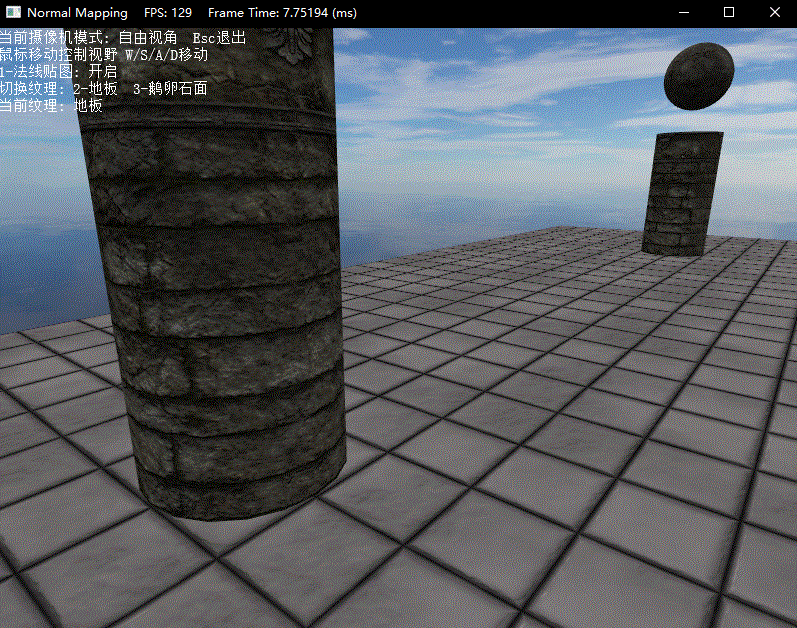DirectX11 With Windows SDK完整目录

Github项目源码

posted @ 2019-01-06 11:17  X_Jun  阅读(2136)  评论(0编辑  收藏  举报
levels of contents